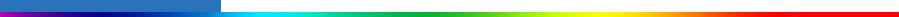Working Group I: The Scientific BasisGet Javascript Other reports in this collection

 Appendix 12.1: Optimal Detection is Regression The detection technique that has been used in most “optimal detection” studies performed to date has several equivalent representations (Hegerl and North, 1997; Zwiers, 1999). It has recently been recognised that it can be cast as a multiple regression problem with respect to generalised least squares (Allen and Tett, 1999; see also Hasselmann, 1993, 1997) in which a field of n “observations” y is represented as a linear combination of signal patterns g1,...,gm plus noise u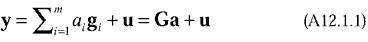where G=(g1|...|gm) is the matrix composed of the signal patterns and a=(a1,...,am)T is the vector composed of the unknown amplitudes. The field usually contains temperature observations, arrayed in space, either at the surface as grid box averages of surface temperature observations (typically 5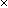5 degrees; Santer et al., 1995; Hegerl et al., 1997; Tett et al., 1999), or in the vertical as zonal averages of radiosonde observations (Karoly et al., 1994; Santer et al., 1996a; Allen and Tett, 1999). The fields are masked so that they represent only those regions with adequate data. The fields may also have a time dimension (Allen and Tett, 1999; North and Stevens; 1998; Stevens and North, 1996). Regardless of how the field is defined, its dimension n (the total number of observed values contained in any one single realisation of the field) is large. The signal patterns, which are obtained from climate models, and the residual noise field, have the same dimension. The procedure consists of efficiently estimating the unknown amplitudes a from observations and testing the null hypotheses that they are zero. In the event of rejection, testing the hypothesis that the amplitudes are unity for some combination of signals performs the attribution consistency test. This assumes, of course, that the climate model signal patterns have been normalised. When the signal is noise-free, estimates of the amplitudes are given by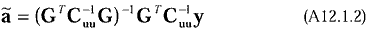where Cuu is the nn covariance matrix of the noise (Hasselmann, 1997, 1998; Allen and Tett, 1999; Levine and Berliner, 1999). Generalisations allow for the incorporation of signal uncertainties (see, for example, Allen et al., 2000b). A schematic two-dimensional example is given in Box 12.1. In essence, the amplitudes are estimated by giving somewhat greater weight to information in the low variance parts of the field of observations. The uncertainty of this estimate, expressed as the mm covariance matrix of Caa of ã, is given by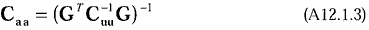This leads to a (1-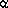)100% confidence ellipsoid for the unknown amplitudes when u is the multivariate Gaussian that is given by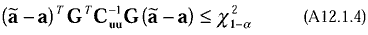where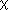21-is the (1-) critical value of the chi-squared distribution with m degrees of freedom. Marginal confidence ellipsoids can be constructed for subsets of signals simply by removing the appropriate rows and columns from GTC-1uuG and reducing the number of degrees of freedom. The marginal (1-)100% confidence interval for the amplitude of signal i (i.e., the confidence interval that would be obtained in the absence of information about the other signals) is given by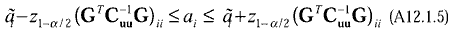where Z1-/2 is the (1-/2) critical value for the standard normal distribution. Signal i is said to be detected at the/2100% significance level if the lower limit confidence interval (A12.1.5) is greater than zero. However, “multiplicity” is a concern when making inferences in this way. For example, two signals that are detected at the/2100% significance level may not be jointly detectable at this level. The attribution consistency test is passed when the confidence ellipsoid contains the vector of units (1,...,1)T.